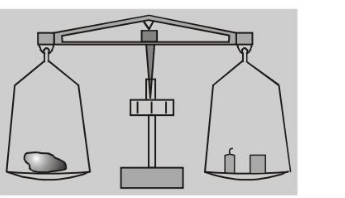# What is Mass?## MASSThe mass of an object is a measure of the object's resistance to a change in its velocity. A child's wagon, coasting along a horizontal sidewalk, is more difficult to stop when it is loaded with bricks than when it is empty. The system, wagon plus load, is more massive in the former case than in the latter. Mass is a scalar quantity and is additive. That is, if we fasten two objects of mass $m_{1}$ and $m_{2}$ together, then the mass $m_{12}$ of the composite system is $m_{12}=m_{1}+m_{2}$

In the laboratory, the mass of an object is often measured with an equal-arm balance by comparison with standard "weights" of known mass. An object's weight is proportional to its mass.

Mass of an object is a property of that object alone.